# Array Basic Operations in C

## Array Basic Operations in C:

In this article, we will discuss how we can perform some basic operations in the given array in C language with Examples. Please read our previous articles where we discussed how we can perform Binary Search for searching an element in a given array in C Language with Examples.

##### Basic Operations in an Array:

Below is the list of functions that we can perform in any array: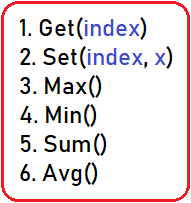Let’s discuss each and every function and see the code syntax:

##### 1. Get (array, index)-

This method will take 2 parameters, the first is the array itself and the second is the index. It will simply return the element which will present at a particular index. If there were no elements or the index will not be a valid index then we will return -1. Let’s we want to find which element is present on index 6 in the following array:At the 6th position in the above array, 24 is present. Let’s take another one, we want to find at index 15, as we can see in the above array, there is no index of 15 so here we have to return -1. So index number should not be less than 0 and not more than the array’s size – 1 (as the array’s indexing starts from 0). We are checking this for the validity of the index. Now, let’s have a look at the code:

##### Array Get() Operation Code in C Language:
```#include<stdio.h>
struct List{
int B;
int size;
int length;
};

int Get(struct List list,int index){
if(index >= 0 && index < list.length)
return list.B[index];
return -1;
}

int main(){
struct List list_1 = {{4, 8, 10, 15, 18, 21, 24, 27, 29, 33, 34, 37, 39, 41, 43},10,9};
int index = 6;
printf("%d is present at index of %d", Get(list_1, index), index);
}
```

Time Complexity: O (1)

Output:##### 2. Set (array, index, x)-

This method will replace the particular element which is present on the given index with the new value of the given element. Below is the array of 15 elements (0 to 14):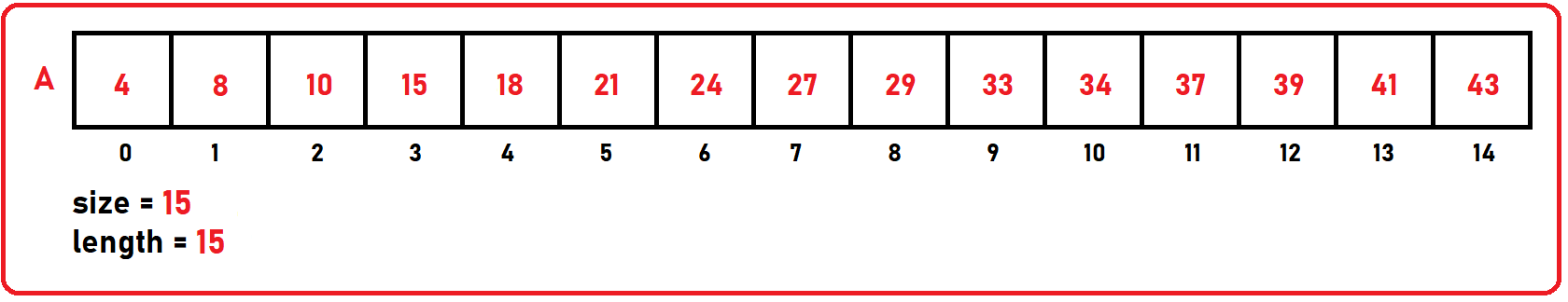Let’s take an example, we want to modify the element which is present on index 8 with the new value of 30, then we will call the set function inside our main function as:

Set (arr, 8, 30);

This method will take 3 parameters- array, index number, and the new value. It is similar to the get function, here also we have to check the validity of the index. The index should not be greater than the array’s size -1 or less than 0. We are using the reference operator in our Set () function so that it can modify the array.

##### Modified Array: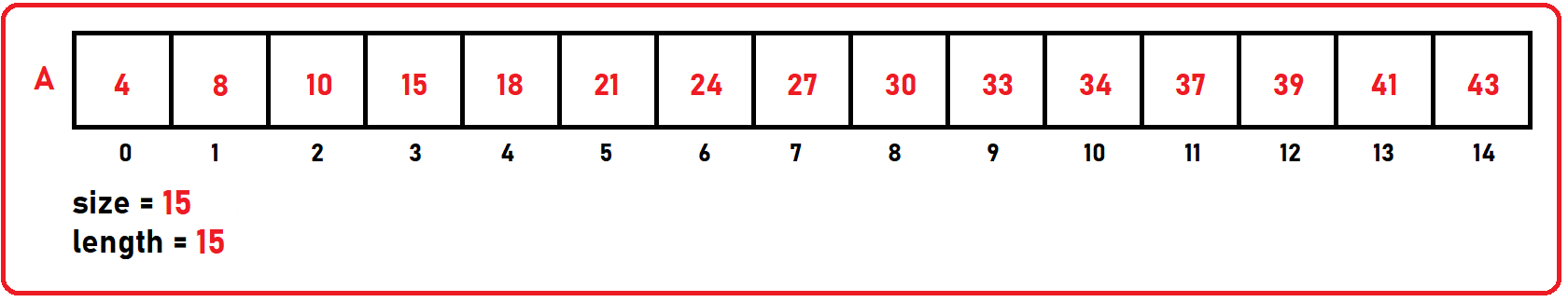Let’s see the code part:

##### Array Set() Operation Code in C Language:
```#include<stdio.h>
struct List{
int B;
int size;
int length;
};

void Set(struct List *list,int index,int x){
if(index >= 0 && index < list->length)
list->B[index] = x;
}

int main(){
struct List list_1 = {{4, 8, 10, 15, 18, 21, 24, 27, 29, 33, 34, 37, 39, 41, 43},10,9};
int index = 8;
int newElement = 30;
Set(&list_1, index, newElement);
printf("%d index is modified with the value of %d", index, newElement);
}
```

Time Complexity: O (1)

Output: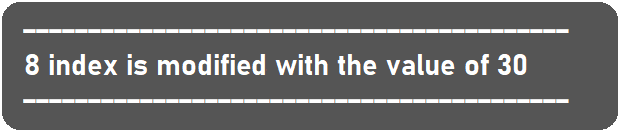##### 3. Max (array)-

This method will take only one parameter which is the array itself. It will return the maximum element present in the array. Below is the array of size 10 (0 to 9).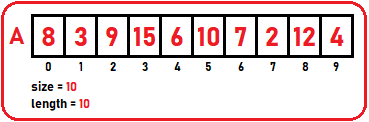Step1: initialize max variable to 0th element of array i.e. max = arr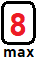Step2: we will iterate through each element of the array and check for if current element > max then max = current element otherwise moves to next element.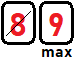min variable keeps modifying until it stores the max element and finally: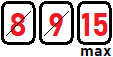As we can see the maximum element present in the above array is 15. So, unless we check the entire array, we cannot say which is the maximum element. If we have a sorted array the last element will be maximum so Time Complexity will be constant, O (1) here. But in the unsorted list, we have to trace the whole array. Then Time Complexity Will be O(n). Let’s see the code part:

##### Array Max() Operation Code in C Language:
```#include<stdio.h>
struct List{
int B;
int size;
int length;
};
int Max(struct List list){
int max = list.B;
for(int i = 1;i < list.length; i++){
if(list.B[i] > max)
max = list.B[i];
}
return max;
}
int main(){
struct List list_1 = {{8, 3, 9, 15, 6, 10, 7, 2, 12, 4},10,9};
printf("%d is max element in the given array", Max(list_1));
}
```

Output: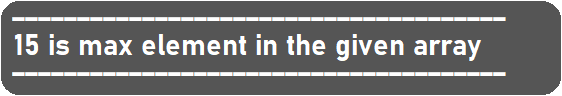##### 4. Min (array)-

This method is the opposite of maximum function. It will take only one parameter i.e. Array and will return the minimum element present in the given array. Below is the array of size 10 (0 to 9).Step1: initialize min variable to 0th element of array i.e. min = arr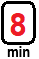Step2: we will iterate through each element of the array and check for if current element < min then min = current element otherwise moves to next element.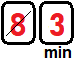min variable keeps modifying until it stores the minimum element and finally: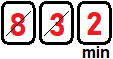As we can see the minimum element present in the above array is 2. So, unless we check the entire array, we cannot say which is the minimum element. If we have a sorted array the 0th element will be minimum so Time Complexity will be constant, O (1) here. But in the unsorted list, we have to trace the whole array. Then Time Complexity will be O (n). Let’s see the code part:

##### Array Min() Operation Code in C Language:
```#include<stdio.h>
struct List{
int B;
int size;
int length;
};
int Min(struct List list){
int min = list.B;
for(int i = 1;i < list.length; i++){
if(list.B[i] < min)
min = list.B[i];
}
return min;
}
int main(){
struct List list_1 = {{8, 3, 9, 15, 6, 10, 7, 2, 12, 4},10,9};
printf("%d is minimum element in the given array", Min(list_1));
}
```

Output: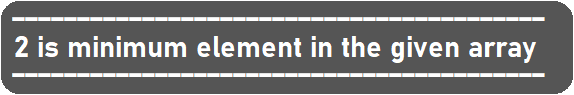##### 5. Sum (array)-

This method will return the sum of all the elements which are present in the given array. For finding the total of all the elements we have to traverse the whole array. Below is the array of size 10 (0 to 9):Step1: First create and initialize a variable “sum” with zero. It holds the total of all elements.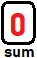Step2: With the help of for loop we will iterate through each element and inside that loop we will add every element to sum variable: i.e. 1st element: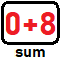Step3: i.e. 2nd element: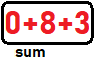Step4: sum of all elements: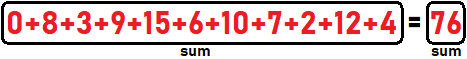At last, we will get the sum of all elements of the array. And then we will return this sum variable from the function.

##### Array Sum() Operation Code in C Language:
```#include<stdio.h>
struct List{
int B;
int size;
int length;
};

int Sum(struct List list){
int sum = 0;
for(int i = 0;i < list.length; i++)
sum+=list.B[i];
return sum;
}

int RSum(struct List list, int n){
if (n < 0)
return 0;
else
return RSum(list, n-1) + list.B[n];
}

int main(){
struct List list_1 = {{8, 3, 9, 15, 6, 10, 7, 2, 12, 4},10,10};
printf("%d is sum of all the element of the given array", Sum(list_1));
}
```

Time Complexity: O (n)

Output: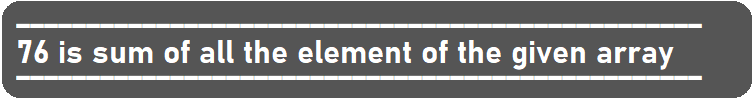##### 6. Average (array)-

This method will return the average of all the elements of the given array. It will take only one parameter: the array itself. Below is the array of size 10 (0 to 9):Step1: Below is the average formula: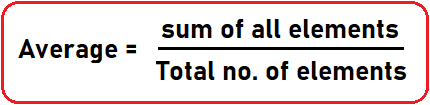Step2: As we have seen the sum() function, we have to modify the return statement as return Total/list.length;

For the above array, the average is: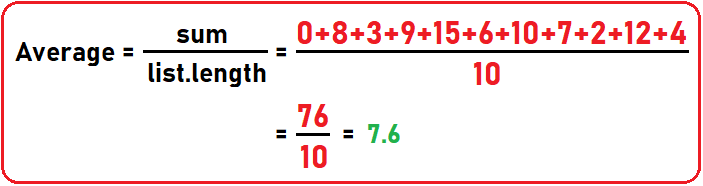Let’s see this in code:

##### Array Average() Operation Code in C Language:
```#include<stdio.h>
struct List{
int B;
int size;
int length;
};

int Sum(struct List list){
int sum = 0;
for(int i = 0;i < list.length; i++)
sum+=list.B[i];
return sum;
}

float Avg(struct List list){
return (float)Sum(list)/list.length;
}

int main(){
struct List list_1 = {{8, 3, 9, 15, 6, 10, 7, 2, 12, 4},10,10};
printf("%f is Average of given array", Avg(list_1));
}
```

Output: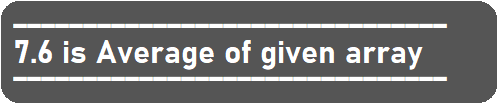In the next article, I am going to discuss Array Reverse and Shift Operations in C Language with Examples. Here, in this article, I try to explain how we can perform some basic operations in the given array with Examples and I hope you enjoy this Array Basic Operations in C Language with Examples article.